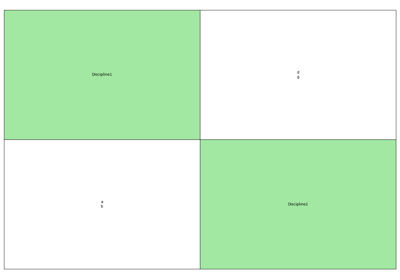gemseo / utils

# study_analysis module¶

Generate a N2 and XDSM into files (and/or web page) from an Excel description of the MDO problem.

class gemseo.utils.study_analysis.StudyAnalysis(xls_study_path)[source]

Bases: object

A MDO study analysis from an Excel specification.

Generate a N2 (equivalent to the Design Structure Matrix) diagram, showing the couplings between the disciplines, and a XDSM (Extended Design Structure Matrix), showing the MDO process, from an Excel specification of the inputs, outputs, design variables, objectives and constraints.

The input Excel files contains one sheet per discipline. The name of the sheet shall have the discipline name. The sheet shall have at least two columns, one for inputs and one for outputs, with the following format:

Disc1

Inputs

Outputs

in1

out1

in2

out2

Empty lines are ignored.

The scenarios (at least one, or multiple for distributed formulations) must appear in a Excel sheet name starting by “Scenario”.

The sheet shall have the following columns, with some constraints :

• All of them are mandatory, even if empty for the constraints.

• The order does not matter.

• One and only one formulation must be declared.

• At least one objective must be provided, and one design variable.

Scenario1

Design variables

Objective function

Constraints

Disciplines

Formulation

Options

Options values

in1

out1

out2

Disc1

MDF

tolerance

0.1

Disc2

main_mda_name

MDAJacobi

All the objective functions and constraints must be outputs of a discipline, not necessarily the one of the current sheet. All the design variables must be inputs of a discipline, not necessarily the one of the current sheet.

The columns ‘Options’ and ‘Options values’ are used to pass the formulation options. Note that for string type ‘Option values’, the value can be written with or without the “” characters.

To use multi level MDO formulations, create multiple scenarios, and add the name of the sub scenarios in the list of disciplines of the main (system) scenario.

An arbitrary number of levels can be generated this way (three, four levels etc formulations).

Initialize the study from the Excel specification.

Parameters:

xls_study_path (str | Path) – The path to the Excel file describing the study.

generate_n2(file_path='n2.pdf', show_data_names=True, save=True, show=False, fig_size=(15, 10))[source]

Generate a N2 plot for the disciplines list.

Parameters:
• file_path (str) –

The file path of the figure.

By default it is set to “n2.pdf”.

• show_data_names (bool) –

If true, the names of the coupling data is shown; otherwise, circles are drawn, which size depends on the number of coupling names.

By default it is set to True.

• save (bool) –

If True, save the figure to file_path.

By default it is set to True.

• show (bool) –

If True, show the plot.

By default it is set to False.

• fig_size (tuple[float, float]) –

The size of the figure.

By default it is set to (15, 10).

Return type:

None

generate_xdsm(output_dir, latex_output=False, open_browser=False)[source]

Create a xdsm.json file from the current scenario.

Parameters:
• output_dir (str) – The directory where the XDSM html files are generated.

• latex_output (bool) –

If True, build the .tex, .tikz and .pdf files.

By default it is set to False.

• open_browser (bool) –

If True, open in a web browser.

By default it is set to False.

Returns:

The MDOScenario that contains the DesignSpace, the formulation, but the disciplines have only correct input and output grammars but no _run methods so that can’t be executed

Return type:

MDOScenario

AVAILABLE_DISTRIBUTED_FORMULATIONS = ('BiLevel', 'BLISS98B')
disciplines: dict[str, MDODiscipline]

The disciplines.

disciplines_descr: dict[str, MDODiscipline]

The descriptions of the disciplines (including sub-scenario) parsed in the Excel file.

scenarios: dict[str, MDOScenario]

The scenarios.

scenarios_descr: dict[str, dict[str, str | list[str]]]

The descriptions of the scenarios parsed in the Excel file.

study: XLSStudyParser

The XLSStudyParser instance built from the Excel file.

xls_study_path: str

The path of the Excel file.

class gemseo.utils.study_analysis.XLSStudyParser(xls_study_path)[source]

Bases: object

Parse the input Excel file that describe the GEMSEO study.

The Excel file must contain one sheet per discipline. The name of the sheet shall have the name of the discipline. The sheet shall have at least two columns, one for the inputs and one for the outputs, with the following format:

Inputs

Outputs

Design variables

Objective function

Constraints

in1

out1

in1

out1

out2

in2

out2

Empty lines are ignored. All the objective functions and constraints must be outputs of a discipline, not necessarily the one of the current sheet. All the design variables must be inputs of a discipline, not necessarily the one of the current sheet.

Initialize the study from the Excel specification.

Parameters:

xls_study_path (str) – The path to the Excel file describing the study.

Raises:
• IOError – If the Excel file cannot be opened.

• ValueError – If no scenario has been found in Excel file.

CONSTRAINTS = 'Constraints'
DESIGN_VARIABLES = 'Design variables'
DISCIPLINE = 'Discipline'
DISCIPLINES = 'Disciplines'
FORMULATION = 'Formulation'
OBJECTIVE_FUNCTION = 'Objective function'
OPTIONS = 'Options'
OPTIONS_VALUES = 'Options values'
SCENARIO_PREFIX = 'Scenario'
disciplines: dict[str, MDODiscipline]

The disciplines.

frames: dict[str, DataFrame]

The data frames created from the Excel file.

inputs: set[str]

The input variables.

outputs: set[str]

The output variables.

scenarios: dict[str, dict[str, str | list[str]]]

The descriptions of the scenarios parsed in the Excel file.

xls_study_path: str

The path to the Excel file.

## Examples using StudyAnalysis¶Generate an N2 and XDSM from an Excel description of the MDO problem

Generate an N2 and XDSM from an Excel description of the MDO problem